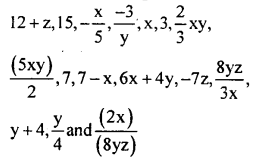# KSEEB Solutions for Class 8 Maths Chapter 2 Algebraic Expressions Ex 2.1

Students can Download Maths Chapter 2 Algebraic Expressions Ex 2.1 Questions and Answers, Notes Pdf, KSEEB Solutions for Class 8 Maths helps you to revise the complete Karnataka State Board Syllabus and score more marks in your examinations.

## Karnataka Board Class 8 Maths Chapter 2 Algebraic Expressions Ex 2.1

Question 1.
Separate the constants and variable from the following.7x + z2,8xy, $$\left(\frac{8}{5}\right)$$x2y2, 4 + 5y – 6z
Monomials: 7 xyz, $$\left(\frac{8}{5}\right)$$ x2y2